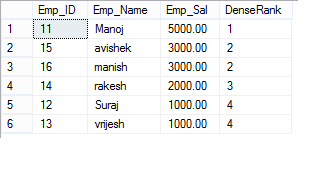#### What is the difference between RANK() and DENSE_RANK() functions in SQL Server .

Total Post:341

Points:2389
1537  View(s)
Ratings:
Rate this:
I want to know What is the difference between RANK() and DENSE_RANK() functions in SQL Server .
please tell me with an example.
1.Post:286

Points:2044
##### Re: What is the difference between the RANK() and DENSE_RANK() functions in SQL Server .

RANK() FUNCTION

The RANK() function will return the ranking of a set of values within a given partition. What exactly do we mean by ranking? Well, suppose we have a set of some values like {3000, 2000, 5000, 1000}. If we arrange those values in order from highest to lowest in descending order, then it would look like {5000, 3000, 2000, 1000}. And if we apply the RANK() function against that set of values, then it will assign each value in the set a “rank”, which is just a numeric integer value starting from 1 that indicates the value’s rank in comparison to the other values in the set. So, the rank for the set {5000, 3000, 2000, 1000} would be {1, 2, 3, 4} – where each rank corresponds to the value in the original set in the same position – so the ranking of 1 is for 5000, 2 is for 3000, etc.

What happens to the rank of a value that comes after a tie? Well, the very next rank would actually skip when using the RANK() function leading to non-consecutive ranks. If that’s confusing, then consider our example set of {3000, 1000, 2000, 3000, 5000, 1000}. Let’s say this set is then sorted in ascending order – so it looks like {1000, 1000, 2000, 3000, 3000, 5000}. The ranking of that set will then be {1, 1, 3, 4, 4, 6}. Note that the “2000” value is assigned a ranking of 3, because a ranking is skipped due to the tie between the two “1000” values that come before. If there were 3 “1000” values in the set, then the rankings would look like {1, 1, 1, 4, 5, 5, 7}, because there is a tie among 3 values, the rank will also skip to a value of 4.

```
SELECT Emp_ID,Emp_Name,Emp_Sal, rank() over (ORDER BY Emp_Sal DESC) AS Ranking
FROM Employee;```DENSE_RANK()

With the DENSE_RANK() function, if there is a tie then none of the ranks will be skipped. This means that the ranks will remain in consecutive order. Let’s take a look at our sample set of values again: {1000, 1000, 2000, 3000, 3000, 5000}. If we apply the DENSE_RANK function against this set of values, then we will end up with these rankings: {1,1,2,3,3,4}. Note that even when there is a tie, the next value will have a ranking that is the next consecutive integer value – and no value will be skipped. And that is why it is called a dense rank – because all the ranking values are used without skipping – maintaining the “density”, or tightness of rank values.

`SELECT Emp_ID,Emp_Name,Emp_Sal,dense_rank() over (ORDER BY Emp_Sal DESC) AS DenseRank FROM Employee;`difference between DENSE_RANK() and RANK()

The one and only difference between the DENSE_RANK() and RANK() functions is the fact that RANK() will assign non-consecutive ranks to the values in a set in the case of a tie, which means that with RANK() there will be gaps between the integer values when there is a tie. But the DENSE_RANK() will assign consecutive ranks to the values in the case of a tie, so there will be no gaps between the integer values in the case of a tie.